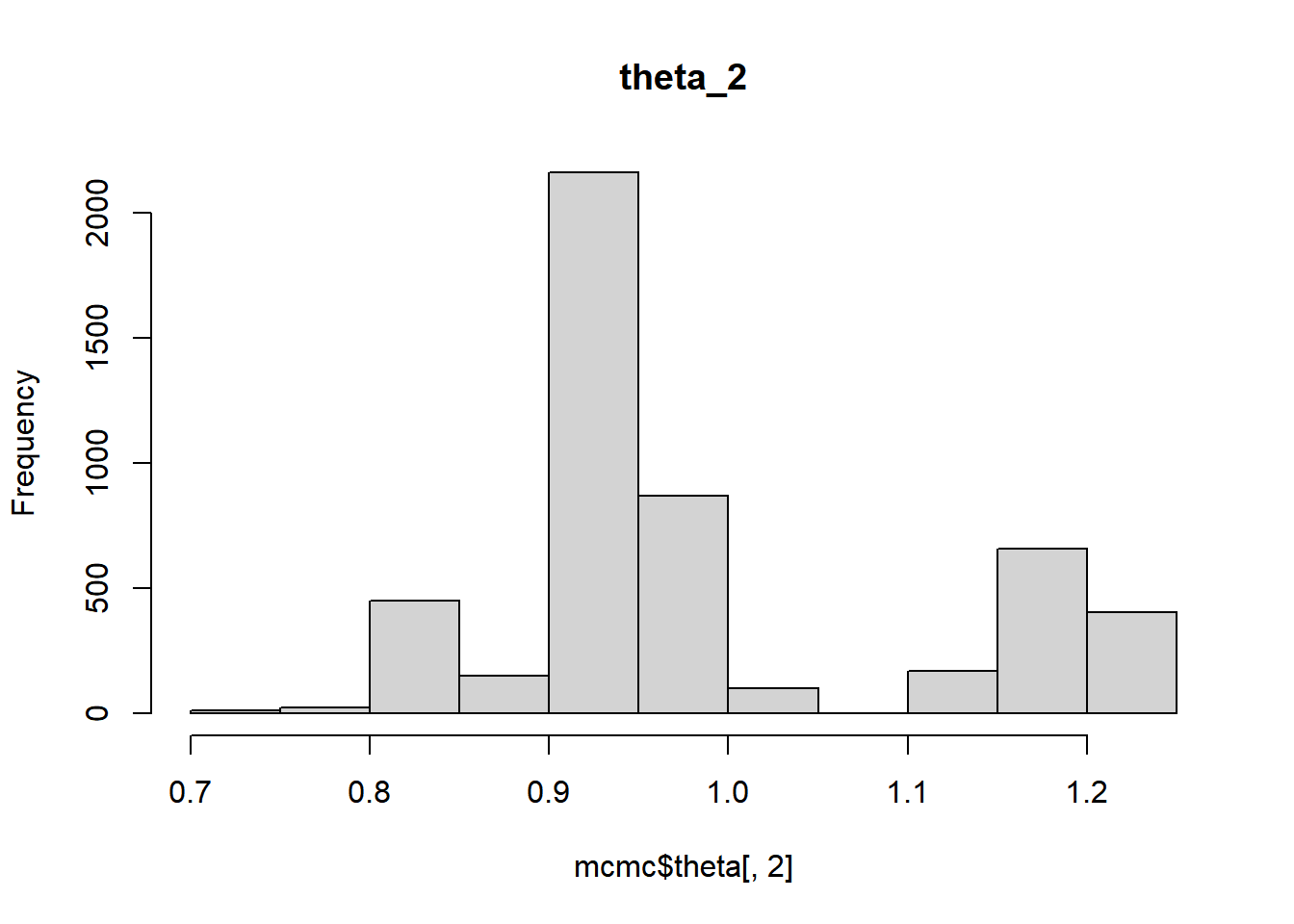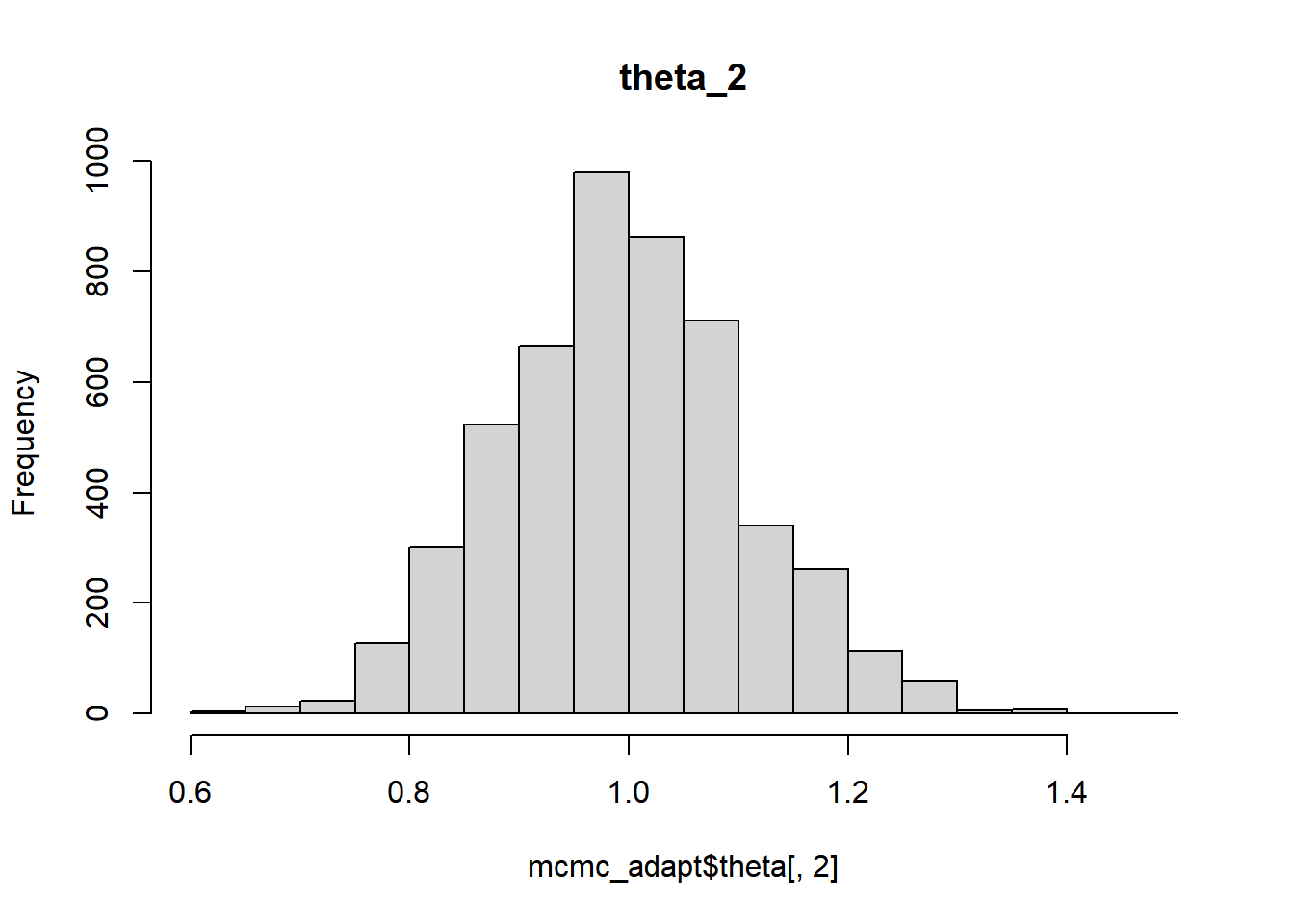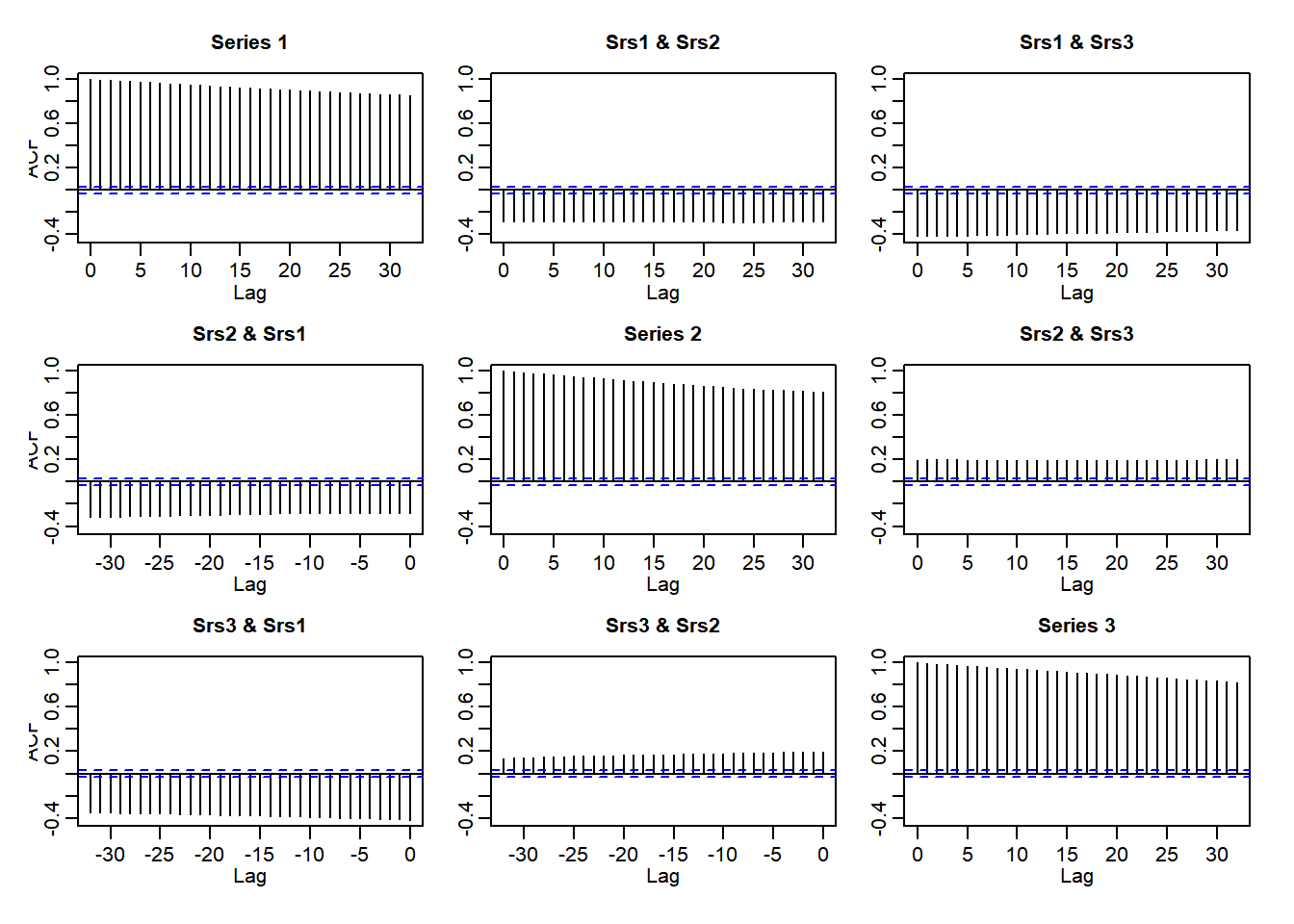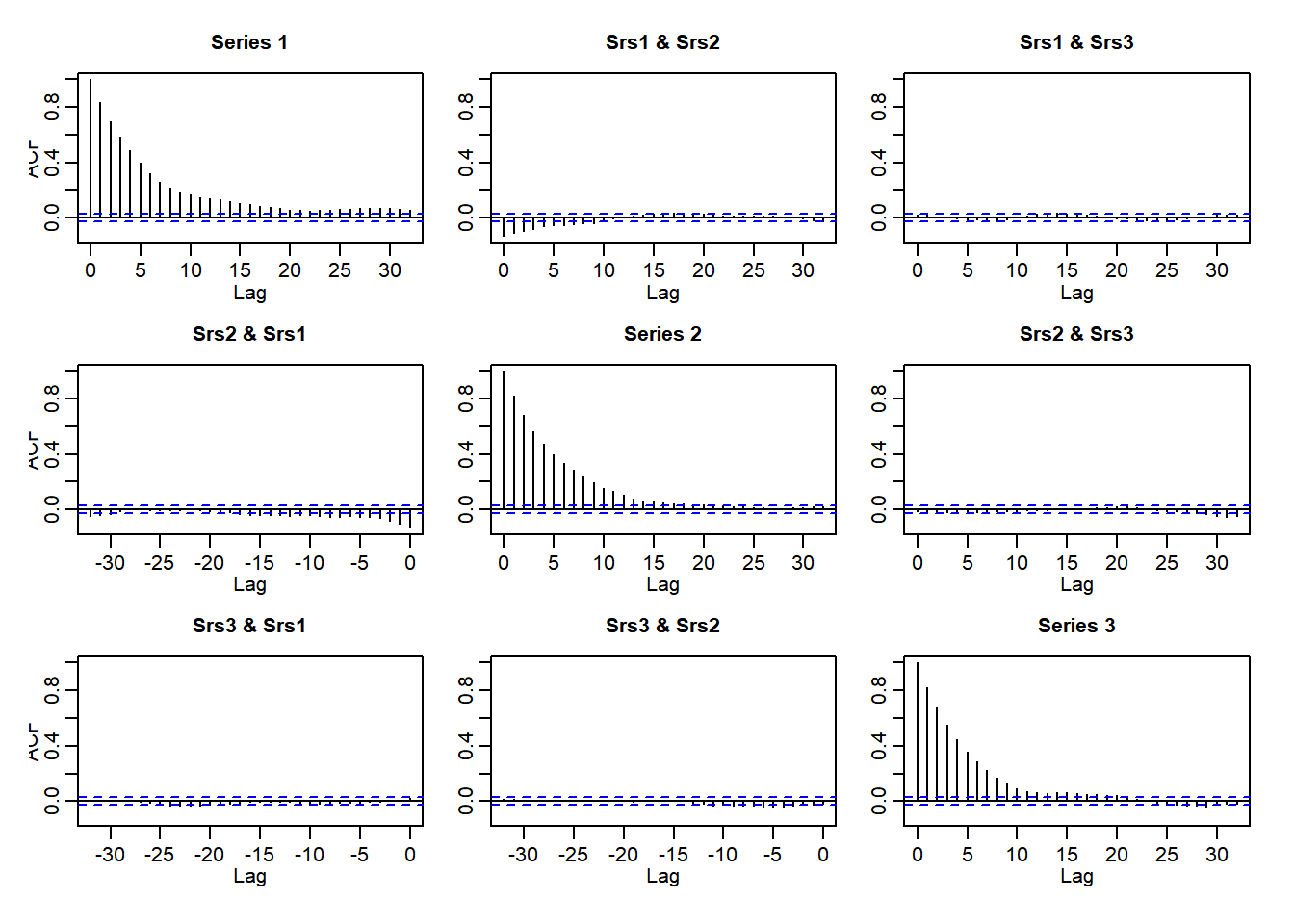# Introduction

This small R package provides key functions for the Robust Adaptive Metropolis (RAM) algorithm by . These functions can be used directly from R or the corresponding header files can be linked to other R packages.

The package cointains three functions, two of which can be useful in more general context as well: Fast Cholesky update and downdate functions, and the actual update function for the scaling matrix of the proposal distribution used in RAM. All functions are written in C++ using Rcpp and RcppArmadillo packages.

# RAM algorithm

The idea of the RAM algorithm is to adaptively update the scaling matrix of the proposal distribution used in the general Metropolis type Markov chain Monte Carlo (MCMC) algoritm. We first fix the target acceptance rate $$\alpha^{\ast}$$, for example to the famous 0.234, and the value of the tuning parameter $$\gamma \in (0,1]$$. Then at iteration $$i$$ of the MCMC:

1. Compute the proposal $$\theta' = \theta_{i-1} + S_{i-1} u_i$$, where $$u_i$$ is a vector of random variable, often from the standard $$d$$-dimensional Gaussian distribution, and $$S_{i-1}$$ is a lower diagonal matrix with positive diagonal elements.
2. Accept the proposal with probability $$\alpha_i := \min\{1, \frac{p(\theta')p(y | \theta')}{p(\theta_{i-1}) p(y | \theta_{i-1})\}}$$.
3. If the proposal $$\theta'$$ is accepted, set $$\theta_i = \theta'$$ Otherwise, set $$\theta_i = \theta_{i-1}$$.
4. Compute the Cholesky factor $$S_i$$ satisfying the equation $S_i S_i^T = S_{i-1}\left(I + \min\{1, d i^{-\gamma}\} (\alpha_i - \alpha^{\ast}) \frac{u_i u_i^T}{\|u_i\|^2}\right) S_{i-1}^T.$

For theoretical details and comparisons with other adaptive Metropolis algorithms, see .

For step (4) of the previous algorithm, instead of computing the right hand side of the equation and performing new Cholesky decomposition, we can use rank-one update or downdate (depending on the sign of $$(\alpha_i - \alpha^{\ast})$$) on the previous $$S_{i-1}$$ in order to obtain new $$S_i$$, which is computationally much more efficient.

## Illustration

As an example, consider a Bayesian estimation of a linear regression model $$y_i = \beta x'_i + \epsilon_i$$, $$\epsilon_i \sim N(0, \sigma^2)$$. For simplicity, we assume non-informative diffuse priors for all model parameters $$(\beta, \sigma)$$, with constraint that $$\sigma$$ must be positive. Let’s first simulate some data:

set.seed(1)
X <- cbind(1, rnorm(100))
theta_true <- c(1, 1, 1)
y <- X %*% theta_true[1:2] + rnorm(100)

And here is our MCMC algorithm which takes in the data (y, X), initial values for the parameters (theta0), initial value for the Cholesky factor for the multivariate normal proposal (S), number of iterations (n_iter), length of the burn-in period (n_burnin), and a logical argument adapt which tells whether we should adapt the proposal covariance or not:

metropolis <- function(y, X, theta0, S, n_iter, n_burnin, adapt = FALSE) {

p <- length(theta0)
theta <- matrix(NA, n_iter, p)
accept <- numeric(n_iter)
mu <- X %*% theta0[1:(p - 1)]
posterior <- sum(dnorm(y, mean = mu, sd = theta0[p], log = TRUE))
theta[1, ] <- theta0

for (i in 2:n_iter){
u <- rnorm(p)
theta_prop <- theta[i - 1, ] + S %*% u
if (theta_prop[p] > 0) {
mu <- X %*% theta_prop[1:(p - 1)]
posterior_prop <- sum(dnorm(y, mean = mu, sd = theta_prop[p], log = TRUE))
acceptance_prob <- min(1, exp(posterior_prop - posterior))
if (runif(1) < acceptance_prob) {
accept[i] <- 1
theta[i, ] <- theta_prop
posterior <- posterior_prop
}else{
theta[i, ] <- theta[i - 1, ]
}
} else {
theta[i, ] <- theta[i - 1, ]
acceptance_prob <- 0
}
if(adapt & i <= n_burnin) {
S <- ramcmc::adapt_S(S, u, acceptance_prob, i - 1)
}
}
list(theta = theta[(n_burnin + 1):n_iter, ], S = S,
acceptance_rate = sum(accept[(n_burnin + 1):n_iter]) / (n_iter - n_burnin))
}

Now we can compare an adaptive and non-adaptive versions:

mcmc <- metropolis(y, X, c(0, 0, 1), diag(1, 3), 1e4, 1e4/2)
mcmc_adapt <- metropolis(y, X, c(0, 0, 1), diag(1, 3), 1e4, 1e4/2, adapt = TRUE)
mcmc$acceptance_rate ##  0.004 mcmc_adapt$acceptance_rate
##  0.2464
mcmc_adapt$S ## [,1] [,2] [,3] ## [1,] 0.16338847 0.00000000 0.0000000 ## [2,] -0.02958480 0.18924912 0.0000000 ## [3,] 0.01159992 0.01019856 0.1243577 hist(mcmc$theta[, 2], main = "theta_2")hist(mcmc_adapt$theta[, 2], main = "theta_2")acf(mcmc$theta)acf(mcmc_adapt\$theta)As we can see, even when we start with a very poor proposal covariance, we get good results with the adaptive MCMC whereas the non-adaptive version is highly inefficient (there are very long autocorrelations present as the acceptance ratio is very small).

# Linking to other R packages

Although one can use functions adapt_S (corresponding to the updating equation of the previous Section), chol_update, and chol_downdate in R, you can also use the underlying C++ code directly in your own R packages on the C++. In order to do so, you just need to add #include "ramcmc.h" to relevant source files in your package, and add ramcmc to LinkingTo field on the DESCRIPTION file of your package.